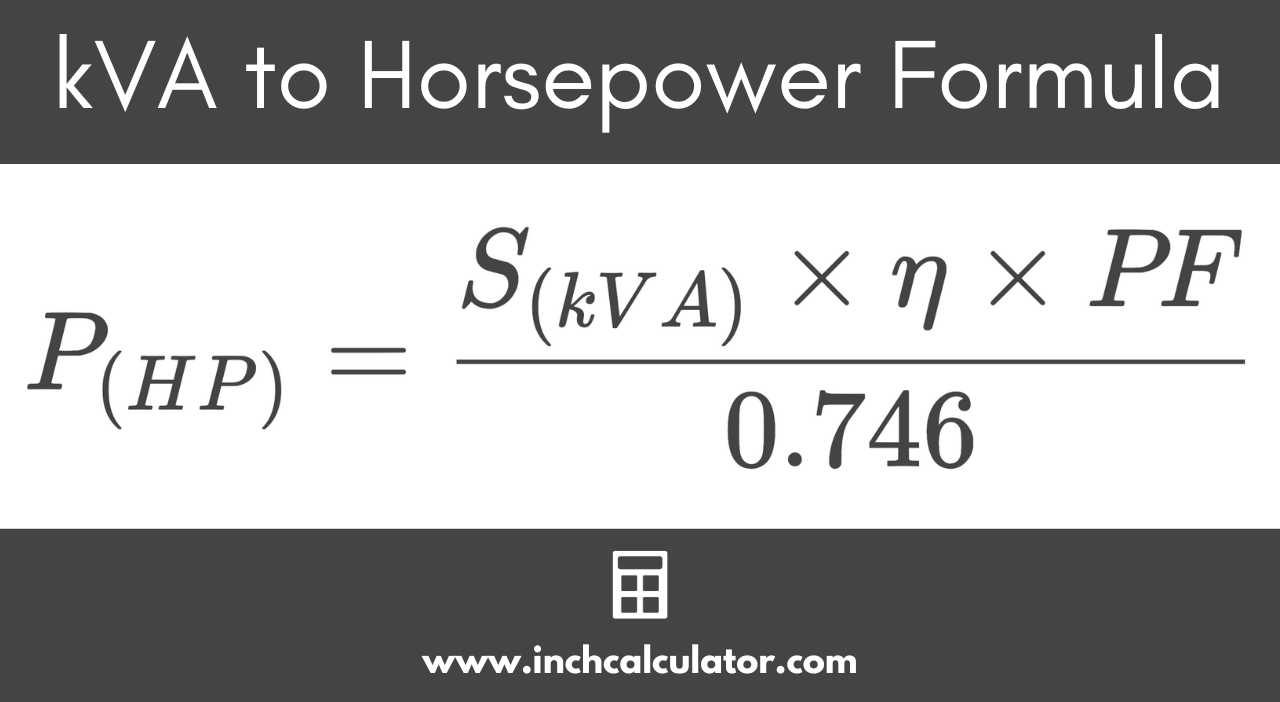# Kilovolt-Amps (kVA) to Horsepower Conversion Calculator

Convert kilovolt-amps (kVA) to horsepower (HP) by entering the kVA, motor efficiency, and power factor below.

kVA
%

## Horsepower Results:

HP
Learn how we calculated this below

## How to Convert kVA to Horsepower

Kilovolt-amps, or kVA, are a measure of the apparent power in a circuit, and horsepower is a measure of electrical output power.

### kVA to Horsepower formula

Because motor efficiency and power factor reduce the amount of apparent power that can be transformed into actual work, the formula below is needed to convert.

P(HP) = S(kVA) × η × PF / 0.746

Thus, the power P in horsepower is equal to the apparent power S in kVA times the motor efficiency η times the power factor PF, divided by 0.746 kW/HP.

For the conversion, replace kVA, efficiency, and power factor values in the formula above, then solve, or just use the calculator above, which does the same thing.To use the formula, follow these two steps.

### Step One: Convert to kW

To convert kVA to horsepower, start by converting kilovolt-amps to kilowatts. Multiply kVA by the power factor, then multiply that by the efficiency if available.

P(kW) = S(kVA) × η × PF

### Step Two: Convert to Horsepower

Finally, divide the kilowatts by 0.746 to get the final result in horsepower.

P(HP) = P(kW) / 0.746

For example, let’s convert 10 kVA to horsepower using a power factor of 0.8 and a motor efficiency of 84%

HP = 10 kVA × 0.84 × 0.8 / 0.746
HP = 6.72 kW / 0.746
HP = 9.008 HP

## Equivalent kVA and Horsepower Ratings

Chart showing kilovolt-amps (kVA) converted to horsepower (HP) ratings, assuming a power factor of 1 and 100% efficiency.
kVA HP
1 kVA 1.34 HP
2 kVA 2.68 HP
3 kVA 4.02 HP
4 kVA 5.36 HP
5 kVA 6.7 HP
6 kVA 8.04 HP
7 kVA 9.38 HP
8 kVA 10.72 HP
9 kVA 12.06 HP
10 kVA 13.4 HP
25 kVA 33.51 HP
50 kVA 67.02 HP
75 kVA 100.5 HP
100 kVA 134 HP
200 kVA 268.1 HP
300 kVA 402.1 HP
400 kVA 536.2 HP
500 kVA 670.2 HP
600 kVA 804.3 HP
700 kVA 938.3 HP
800 kVA 1,072 HP
900 kVA 1,206 HP
1,000 kVA 1,340 HP
2,000 kVA 2,681 HP
3,000 kVA 4,021 HP
4,000 kVA 5,362 HP
5,000 kVA 6,702 HP
6,000 kVA 8,043 HP
7,000 kVA 9,383 HP
8,000 kVA 10,724 HP
9,000 kVA 12,064 HP
10,000 kVA 13,405 HP

Do you want to convert horsepower to kVA?